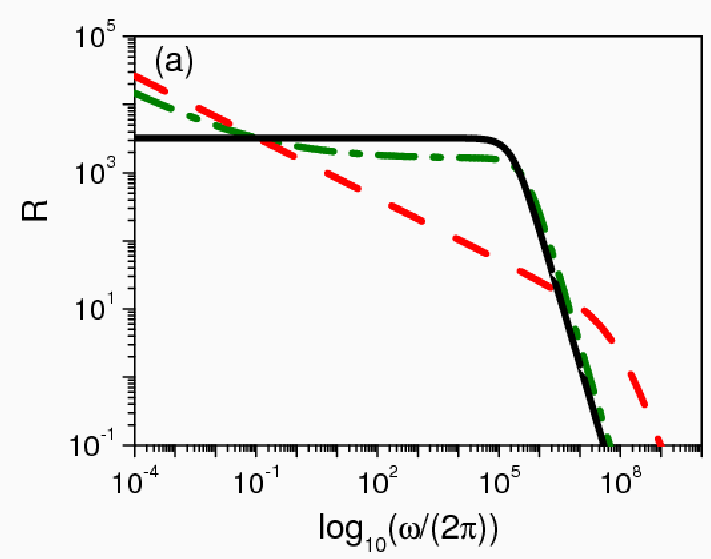# Fractional Calculus in Electrical Impedance Spectroscopy: Poisson-Nernst-Planck model and Extensions### Abstract

We review some analytical results obtained in the context of the fractional calculus for the electrical spectroscopy impedance, a technique usually employed to interpret experimental data regarding the electrical response of an electrolytic cell. We start by reviewing the main points of the standard Poisson – Nernst – Planck model. After, we present an extension that incorporates fractional time derivatives of distributed order to the diffusion equation. Then, we include fractional time derivatives on the boundary conditions in order to face the problems that are characterized, in the low frequency limit, by a frequency dispersion and, consequently, leads to a response in the form , where is the electrical impedance, , with being the frequency of the applied voltage, and . This scenario is extended in order to encompass also the systems characterized by Ohmic electrodes. For these cases, by focusing the low frequency regime, we discuss the applicability of such extensions as a tool to describe experimental data. This analysis is applied in the description of the electrical impedance of electrolytic cells with Milli – Q water and a weak electrolytic solution of KCl.

Type